Next: Unitarity Limit Up: Examples Previous: Examples

The hadronic current can be divided in one part corresponding to conservation of strageness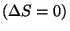and in another which changes strangeness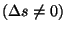: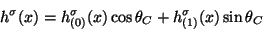(83)

where the mixing angle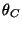is constant and is called Cabbibo angle. This angle was introduced in order to take into account the fact that the reactions with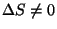have much less amplitude than the ones preserving strtangeness. Universality in the hadronic world is then written: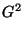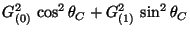(84)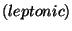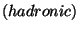(85)

The phenomenology indicates that each one of the parts of (85) has a vectorial and an axial component, namely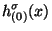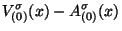(86)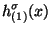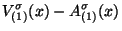(87)

that is, has the structure (V-A) as in the leptonic case.

As a complete theory of the strong interactions of hadrons is not avaliable, it is not possible to give a simple expression of the hadronic current in terms of operators for hadrons. Nevertheless we can go deeper in the level of elementarity and take the quark model (then it will be the basis of the quark theory : QCD). The model of quarks comes from the hypothesis that the hadrons are bound states of quarks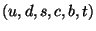.

In order to present the usual phenomenology we can restrict ourselves to a model with only light quarks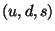. We have then a unitary internal symmetry of flavor,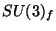. Under this symmetry, the quark triplet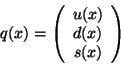(88)

is transformed as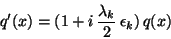(89)

where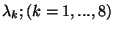are the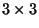matrices of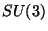. If the masses of quarks are equal, the equation of motion of the free quarks is: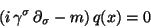(90)

It is then found a conserved vector current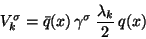(91)

and a non conserved axial current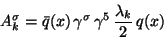(92)

because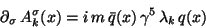(93)

In terms of the currents so defined, the electromagnetic current is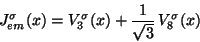(94)

meanwhile the weak components with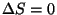and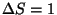are: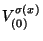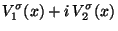(95)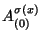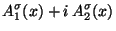(96)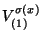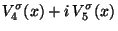(97)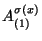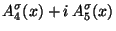(98)

The Cabbibo hypothesis for the hadronic current is written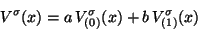(99)

with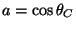and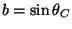. Due to the fact that the only invariant under SU(3) is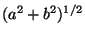, it results that the condition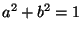(Cabbibo universality) is also invariant, but asis experimentally fixed in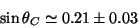(100)

one inmediatelly concludes that there is a breaking of.Next: Unitarity Limit Up: Examples Previous: Examples
root 2001-01-22